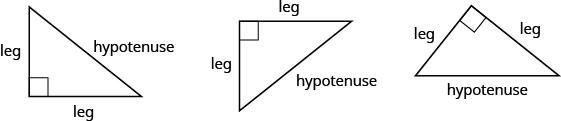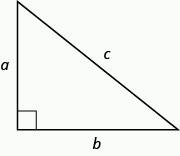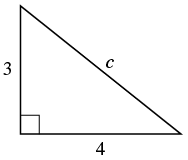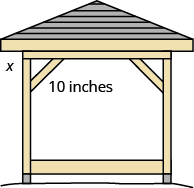## Using the Pythagorean Theorem to Solve Problems

### Learning Outcomes

• Use the Pythagorean theorem to find the unknown side length of a right triangle

The Pythagorean theorem is a special property of right triangles that has been used since ancient times. It is named after the Greek philosopher and mathematician Pythagoras who lived around $500$ BCE.

Remember that a right triangle has a $90^\circ$ angle, which we usually mark with a small square in the corner. The side of the triangle opposite the $90^\circ$ angle is called the hypotenuse, and the other two sides are called the legs. See the triangles below.

In a right triangle, the side opposite the $90^\circ$ angle is called the hypotenuse and each of the other sides is called a leg.The Pythagorean theorem tells how the lengths of the three sides of a right triangle relate to each other. It states that in any right triangle, the sum of the squares of the two legs equals the square of the hypotenuse.

### The Pythagorean Theorem

In any right triangle $\Delta ABC$,

${a}^{2}+{b}^{2}={c}^{2}$

where $c$ is the length of the hypotenuse $a$ and $b$ are the lengths of the legs.To solve problems that use the Pythagorean theorem, we will need to find square roots. Previously, we introduced the notation $\sqrt{m}$ and defined it in this way:

$\text{If }m={n}^{2},\text{ then }\sqrt{m}=n\text{ for }n\ge 0$

For example, we found that $\sqrt{25}$ is $5$ because ${5}^{2}=25$.

We will use this definition of square roots to solve for the length of a side in a right triangle.

### example

Use the Pythagorean theorem to find the length of the hypotenuse.Solution

 Step 1. Read the problem. Step 2. Identify what you are looking for. the length of the hypotenuse of the triangle Step 3. Name. Choose a variable to represent it. Let $c=\text{the length of the hypotenuse}$Step 4. Translate. Write the appropriate formula. Substitute. ${a}^{2}+{b}^{2}={c}^{2}$ ${3}^{2}+{4}^{2}={c}^{2}$ Step 5. Solve the equation. $9+16={c}^{2}$ $25={c}^{2}$ $\sqrt{25}={c}$ $5=c$ Step 6. Check: ${3}^{2}+{4}^{2}=\color{red}{{5}^{2}}$ $9+16\stackrel{?}{=}25$ $25+25\checkmark$ Step 7. Answer the question. The length of the hypotenuse is $5$.

### example

Use the Pythagorean theorem to find the length of the longer leg.### example

Kelvin is building a gazebo and wants to brace each corner by placing a $\text{10-inch}$ wooden bracket diagonally as shown. How far below the corner should he fasten the bracket if he wants the distances from the corner to each end of the bracket to be equal? Approximate to the nearest tenth of an inch.### try it

In the following video we show two more examples of how to use the Pythagorean theorem to solve application problems.

## Contribute!

Did you have an idea for improving this content? We’d love your input.